# 基于本证间隙增大的K邻域加权谱聚类算法K Neighbor Relationship for Spectral Clustering with Lager Eigengap

DOI: 10.12677/CSA.2020.102030, PDF, HTML, XML, 下载: 264  浏览: 496

Abstract: Recently, clustering is one of the hot method in unsupervised learning. Spectral clustering is a hot in clustering method and often shows good clustering performance. One crucial step of spectral clustering is constructing a similarity matrix. However, the traditional model cannot consider the distribution structure of the data set well, and it is diﬃcult to truly reﬂect the similarity between data points. Inspired by density clustering to ﬁnd the nearest neighbor relationship between data and obtain the optimized similarity matrix. In this paper, we propose a novel construction method of similarity matrix that use a graph with kneighbor relationship (KNRS), considering the kneighborhood distribution of data this model, as a result, the eigengap becomes lager. We have also made comparison with some methods on some common datasets, the experiments show the superiority of our model in the benchmark data sets.

1. 引言

2. 谱聚类算法及分析

2.1. 谱聚类

2.2. 相似度矩阵

2.3. 本征间隙

3. KN-SC算法及证明

3.1. 概念定义

$d\left(i,j\right)=\sqrt{{\left({x}_{i1}-{x}_{j1}\right)}^{2}+{\left({x}_{i2}-{x}_{j2}\right)}^{2}+\cdots +{\left({x}_{in}-{x}_{jn}\right)}^{2}}$ (1)

$A\left(i,j\right)=\left\{\begin{array}{l}1;\text{\hspace{0.17em}}\text{\hspace{0.17em}}\text{ }\text{if}\text{\hspace{0.17em}}j\text{th point distance}\le k\text{th}\text{\hspace{0.17em}}\text{points distance}\\ 0;\text{\hspace{0.17em}}\text{\hspace{0.17em}}\text{if}\text{\hspace{0.17em}}j\text{th point distance}>k\text{th points distance}\end{array}$ (2)

k是KNRS的参数，k用于找出每个点的k个最近邻。

$\rho =\mathrm{exp}\left(-x+\mathrm{log}\left(1-\alpha \right)\right)$ (3)

$x=\left({\sum }_{i=1}^{n}A\right)/k$ (4)

$\delta =\mathrm{min}\left\{|\lambda -s|;\lambda \text{\hspace{0.17em}}\text{eigenvalue}\text{\hspace{0.17em}}\text{of}\text{\hspace{0.17em}}A,\lambda \notin {S}_{1},s\in {S}_{1}\right\}$ (5)

${V}_{1}$${\stackrel{˜}{V}}_{1}$ 之间的距离 $d\left({V}_{1},{\stackrel{˜}{V}}_{1}\right)$ 的范围为：

$d\left({V}_{1},{\stackrel{˜}{V}}_{1}\right)\le \frac{‖H‖}{\delta }$ (6)

3.2. KNRS算法

KNRS方法包括五个阶段，大致流程如图1所示。

$L=D-W$ (7)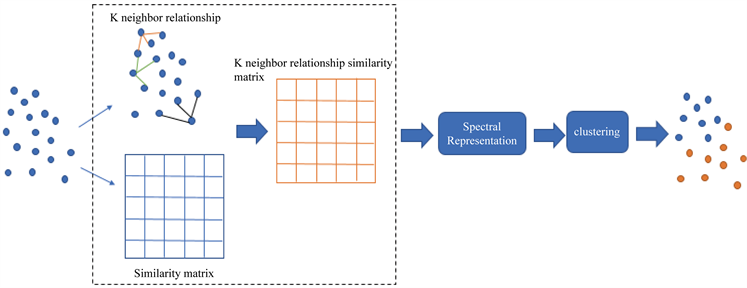Figure 1. KNRS algorithm flowchart

${L}_{sym}={D}^{-1/2}L{D}^{1/2}=I-{D}^{\frac{-1}{2}}W{D}^{\frac{1}{2}}$ (8)

${L}_{rw}={D}^{-1}L=I-{D}^{-1}W$. (9)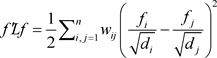(10)

$\begin{array}{c}{f}^{\text{T}}Lf={f}^{\text{T}}\left(I-{D}^{-\frac{1}{2}}W{D}^{-\frac{1}{2}}\right)f\\ =\underset{i=1}{\overset{n}{\sum }}{f}_{i}^{2}-\underset{i,j=1}{\overset{n}{\sum }}{f}_{i}{f}_{j}\frac{{w}_{ij}}{\sqrt{{d}_{i}{d}_{j}}}\\ =\frac{1}{2}\left(\underset{i=1}{\overset{n}{\sum }}{f}_{i}^{2}+\underset{j=1}{\overset{n}{\sum }}{f}_{j}^{2}-2\underset{i,j=1}{\overset{n}{\sum }}{f}_{i}{f}_{j}\frac{{w}_{ij}}{\sqrt{{d}_{i}{d}_{j}}}\right)\\ =\frac{1}{2}{\underset{i,j=1}{\overset{n}{\sum }}{w}_{ij}\left(\frac{{f}_{i}}{\sqrt{{d}_{i}}}-\frac{{f}_{j}}{\sqrt{{d}_{j}}}\right)}^{2}\end{array}$ (11)

1. 第一步，构建完整的连接图并选择最近的k个点，获得k个最近的邻居矩阵W。

2. 第二步，找到k邻居关系。

3. 第三步，获得新的相似矩阵W。

4. 第四步，获得拉普拉斯矩阵L。

5. 第五步，使用例如K-均值算法得到最终的聚类结果。

3.3. KNRS算法相关证明

$f\left(D-W\right)\ge f\left({e}_{\mathrm{min}}I-W\right)~{e}_{\mathrm{min}}-\lambda \ge {e}_{\mathrm{min}}-{\lambda }_{\mathrm{max}}\ge {e}_{\mathrm{min}}-\mathrm{min}\left({‖W‖}_{\infty },{‖W‖}_{1}\right)$ (12)

${\mathrm{max}}_{1\le i\le n}|{\lambda }_{i}|\le {‖A‖}_{1}={\mathrm{max}}_{1\le j\le n}{\sum }_{i=1}^{n}|{a}_{ij}|$ (13)

${\mathrm{max}}_{1\le i\le n}|{\lambda }_{i}|\le {‖A‖}_{\infty }={\mathrm{max}}_{1\le i\le n}{\sum }_{j=1}^{n}|{a}_{ij}|$ (14)

$f\left(D-W\right)\le f\left({e}_{\mathrm{max}}I-W\right)~{e}_{\mathrm{max}}-\lambda \le {e}_{\mathrm{max}}-{\lambda }_{\mathrm{min}}\le \mathrm{min}\left({‖W‖}_{\infty },{‖W‖}_{1}\right)-{\lambda }_{\mathrm{min}}$ (15)

$\begin{array}{c}\Delta =sup\left(L\right)-sub\left(L\right)\\ =\left(\mathrm{min}\left({‖W‖}_{\infty },{‖W‖}_{1}\right)-{\lambda }_{\mathrm{min}}\right)-\left({e}_{\mathrm{min}}-\mathrm{min}\left({‖W‖}_{\infty },{‖W‖}_{1}\right)\right)\\ =-{\lambda }_{\mathrm{min}}-{e}_{\mathrm{min}}+2\mathrm{min}\left({‖W‖}_{\infty },{‖W‖}_{1}\right)\\ =\mathrm{min}\left({‖W‖}_{\infty },{‖W‖}_{1}\right)-{\lambda }_{\mathrm{min}}\text{\hspace{0.17em}}\left(\text{assym}\text{.matrix}W\right)\\ \le {\lambda }_{\mathrm{max}}-{\lambda }_{\mathrm{min}}\le {\lambda }_{\mathrm{max}}\end{array}$ (16)

$\begin{array}{c}\Delta =sup\left(L\right)-sub\left(L\right)\\ \le {\lambda }_{\mathrm{max}}\\ \le ‖L+{H}^{\prime }‖\\ \le ‖L‖+‖{H}^{\prime }‖\end{array}$ (17)

4. 实验

4.1. 数据集

Iris数据集包含3个类别，每个类别有50个实例，具有4个属性，其中每个类别都表示一种Iris植物。Wine数据集包含三种葡萄酒中178种样品中每种的13种成分的数量。Soybean数据集有47个样本，35个属性和7个类别。Seeds数据集具有210个样本，7个属性和3个类别。Two circles的人工数据集在两个圆圈中具有420个样本，在两个类别中具有三个属性。Boat数据集包含100个样本，2个属性和3个类别。这两个人工数据集包含两种类型的样本，即中等密度密集区域和周围稀疏区域。MNIST手写数字数据库的训练集为60,000个示例，测试集为10,000个示例，784个属性和10个类别用来验证算法不仅对密度均匀的数据集聚类有效，而且对于密度分布不均匀的数据集聚类也有效。标准数据集用于进一步检查算法的有效性和泛化能力。

4.2. 聚类评价指标

4.2.1. NMI

$NMI=\frac{I\left(H,Y\right)}{\sqrt{H\left(X\right)H\left(Y\right)}}$ (18)

$NMI=\frac{{\sum }_{k=1}^{C}{\sum }_{m=1}^{C}{n}_{k,m}\mathrm{log}\left(\frac{n×{n}_{k,m}}{{n}_{k}{\stackrel{^}{n}}_{m}}\right)}{\sqrt{\left({\sum }_{k=1}^{C}{n}_{k}\mathrm{log}\frac{{n}_{k}}{n}\right)\left({\sum }_{m=1}^{C}{\stackrel{^}{n}}_{m}\mathrm{log}\frac{{\stackrel{^}{n}}_{m}}{n}\right)}}$ (19)

4.3. 实验总结分析

4.3.1. 章节标题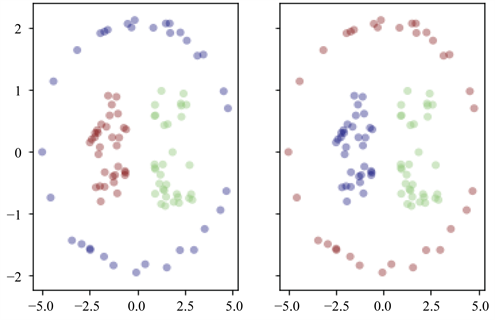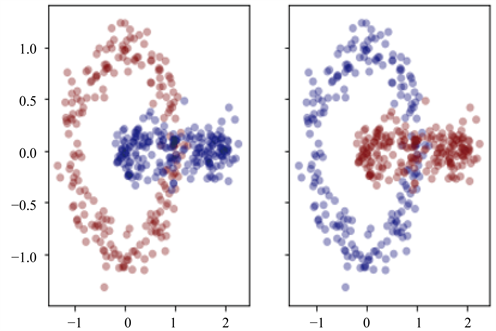Figure 2. KNRS clustering results on benchmark datasetsTable 2. Results on datasets by NMI

MNIST是手写数字数据库如图3所示，对于那些想在真实数据上尝试学习和模式识别方法同时又在预处理和格式化上花费最少精力的人们来说，是一个很好的数据库。本文从MNIST的训练集中选择了500个图像中的150个属性进行实验。NMI对MNIST数据集的聚类结果总结在图4中。根据图4，我们可以看到KNRS方法优于其他比较方法。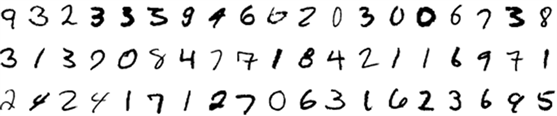Figure 3. MNIST dataset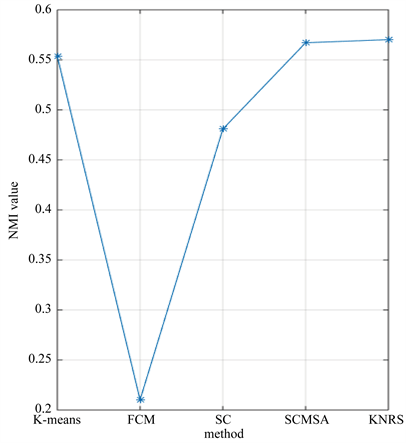Figure 4. Results on MNIST datasets by NMI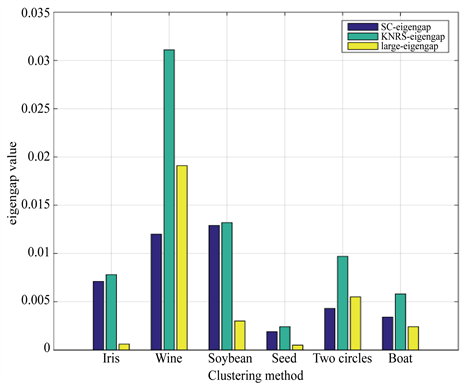Figure 5. Results of increased eigengap of dataset in KNRS and SC methods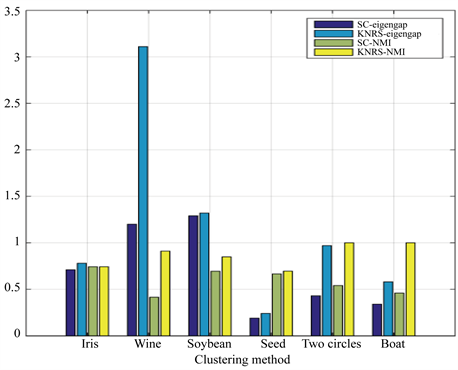Figure 6. Comparison of the effect of increasing the eigenspace on NMI on 6 sets of datasets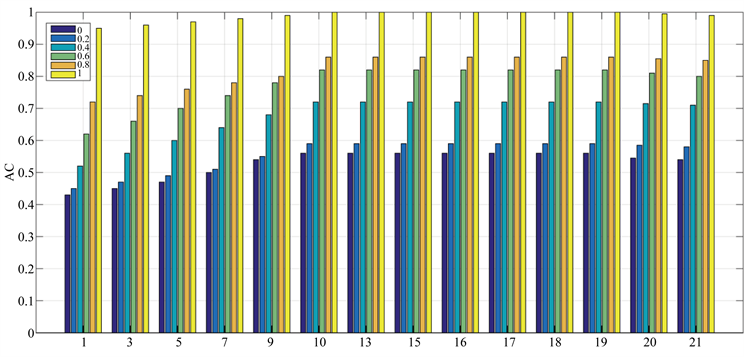Figure 7. Clustering results of KNRS method for different δ and k. (δ = 0.1, 0.2, 0.4, 0.8, 1); number of neighbors k = 1, 3, 5, 7, 9, 10, 13, 15, 16, 17, 18, 19, 20, 21

5. 总结

  Jain, A.K. (2008) Data Clustering: 50 Years beyond K-Means.  Park, H.S. and Jun, C.H. (2009) A Simple and Fast Algorithm for k-Medoids Clustering. Expert Systems with Applications, 36, 3336-3341. https://doi.org/10.1016/j.eswa.2008.01.039  Guha, S., Rastogi, R. and Shim, K. (1998) Cure: An Efficient Clustering Algorithm for Large Databases. Information Systems, 26, 35-58. https://doi.org/10.1016/S0306-4379(01)00008-4  Bezdek, J.C., Ehrlich, R. and Full, W. (1984) FCM: The Fuzzy C-Means Clustering Algorithm. Computers & Geosciences, 10, 191-203. https://doi.org/10.1016/0098-3004(84)90020-7  Ester, M., Kriegel, H.P., Sander, J., et al. (1996) A Density-Based Algorithm for Discovering Clusters in Large Spatial Databases with Noise. Proceedings of International Conference on Knowledge Discovery & Data Mining, Vol. 96, 226-231.  Luxburg, U.V. (2007) A Tutorial on Spectral Clustering. Statistics & Computing, 17, 395-416. https://doi.org/10.1007/s11222-007-9033-z  Schlkopf, B., Smola, A. and Mller, K. (1998) Nonlinear Component Analysis as a Kernel Eigen Value Problem. Neural Computation, 10, 1299-1319.  Xu, D. and Tian, Y. (2015) A Comprehensive Survey of Clustering Algorithms. Annals of Data Science, 2, 165-193. https://doi.org/10.1007/s40745-015-0040-1  Donath, W.E. and Hoﬀman, A.J. (1973) Lower Bounds for the Partitioning of Graphs. IBM Journal of Research & Development, 17, 420-425. https://doi.org/10.1147/rd.175.0420  Fiedler, M. (1976) Algebraic Connectivity of Graphs. Czechoslovak Mathematical Journal, 23, 298-305.  Hagen, L. and Kahng, A.B. (2002) New Spectral Methods for Ratio Cut Partitioning and Clustering. IEEE Transactions on Computer-Aided Design of Integrated Circuits and Systems, 11, 1074-1085. https://doi.org/10.1109/43.159993  Shi, J. and Malik, J. (2000) Normalized Cuts and Image Segmentation. Departmental Papers (CIS), 107.  Ng, A.Y., Jordan, M.I. and Weiss, Y. (2002) On Spectral Clustering: Analysis and an Algorithm. In: Advances in Neural Information Processing Systems, Springer, The Netherlands, 849-856.  Feng, J., Lin, Z., Xu, H. and Yan, S. (2014) Robust Subspace Segmentation with Block-Diagonal Prior. 2014 IEEE Conference on Computer Vision and Pattern Recognition, Columbus, OH, 23-28 June 2014, 3818-3825. https://doi.org/10.1109/CVPR.2014.482  Xia, T., Cao, J., Zhang, Y.-D. and Li, J.-T. (2009) On Defining Affinity Graph for Spectral Clustering through Ranking on Manifolds. https://doi.org/10.1016/j.neucom.2009.03.012  Zhang, X., Li, J. and Yu, H. (2011) Local Density Adaptive Similarity Measurement for Spectral Clustering. Pattern Recognition Letters, 32, 352-358. https://doi.org/10.1016/j.patrec.2010.09.014  孙继广. 矩阵扰动分析[M]. 北京: 科学出版社, 2001: 146-160.  Davis, C. and Kahan, W.M. (1970) The Rotation of Eigenvectors by a Perturbation. SIAM Journal on Numerical Analysis, 7, 1-46. https://doi.org/10.1137/0707001  Perona, P. and Freeman, W. (1998) A Factorization Approach to Grouping. In: European Conference on Computer Vision, Springer, Netherlands, 655-670. https://doi.org/10.1007/BFb0055696  Scott, G.L. and Longuet-Higgins, H.C. (1990) Feature Grouping by ‘Relocalisation’ of Eigenvectors of the Proximity Matrix. BMVC, 1-6. https://doi.org/10.5244/C.4.20  Ding, C.H., He, X., Zha, H., Gu, M. and Simon, H.D. (2001) A Min-Max Cut Algorithm for Graphpartitioning and Data Clustering. Proceedings 2001 IEEE International Conference on Data Mining, San Jose, CA, 29 November-2 December 2001, 107-114.  王丽. 图论在算法设计中的应用[D]: [硕士学位论文]. 西安: 西安电子科技大学, 2010.  Malik, J., Belongie, S., Leung, T. and Shi, J. (2001) Contour and Texture Analysis for Image Segmentation. International Journal of Computer Vision, 43, 7-27.  Meila, M. and Shi, J. (2001) Learning Segmentation by Random Walks. In: Advances in Neural Information Processing Systems, Springer, The Netherlands, 873-879.  Liu, R., Lin, Z., and Su, Z. (2014) Learning Markov Random Walks for Robust Subspace Clustering and Estimation. Neural Networks, 59, 1-15. https://doi.org/10.1016/j.neunet.2014.06.005  Li, X.-Y. and Guo, L.-J. (2012) Constructing Affinity Matrix in Spectral Clustering Based on Neighbor Propagation. Neurocomputing, 97, 125-130. https://doi.org/10.1016/j.neucom.2012.06.023  Li, Q., Ren, Y., Li, L. and Liu, W. (2016) Fuzzy Based Affinity Learning for Spectral Clustering. Pattern Recognition, 60, 531-542. https://doi.org/10.1016/j.patcog.2016.06.011  田铮, 李小斌, 句彦伟. 谱聚类的扰动分析[J]. 中国科学, 2007, 37(4): 527-543.  孔万增, 孙志海, 杨灿. 基于本征间隙与正交特征向量的自动谱聚类[J]. 电子学报, 2010, 38(8): 1980-1985.  Bhatia, R. (2013) Matrix Analysis. Springer Science & Business Media, New York.  He, X., Cai, D. and Niyogi, P. (2006) Laplacian Score for Feature Selection. In: Advances in Neural Information Processing Systems, Springer, The Netherlands, 507-514.  Xiang, T. and Gong, S. (2008) Spectral Clustering with Eigenvector Selection. Pattern Recognition, 41, 1012-1029. https://doi.org/10.1016/j.patcog.2007.07.023  Asuncion, A. and Newman, D. (2007) UCI Machine Learning Repository.  Strehl, A. and Ghosh, J. (2002) Cluster Ensembles—A Knowledge Reuse Framework for Combining Multiple Partitions. Journal of Machine Learning Research, 3, 583-617.  Jin, R., Kang, F. and Ding, C.H. (2006) A Probabilistic Approach for Optimizing Spectral Clustering. In: Advances in Neural Information Processing Systems, Springer, The Netherlands, 571-578.